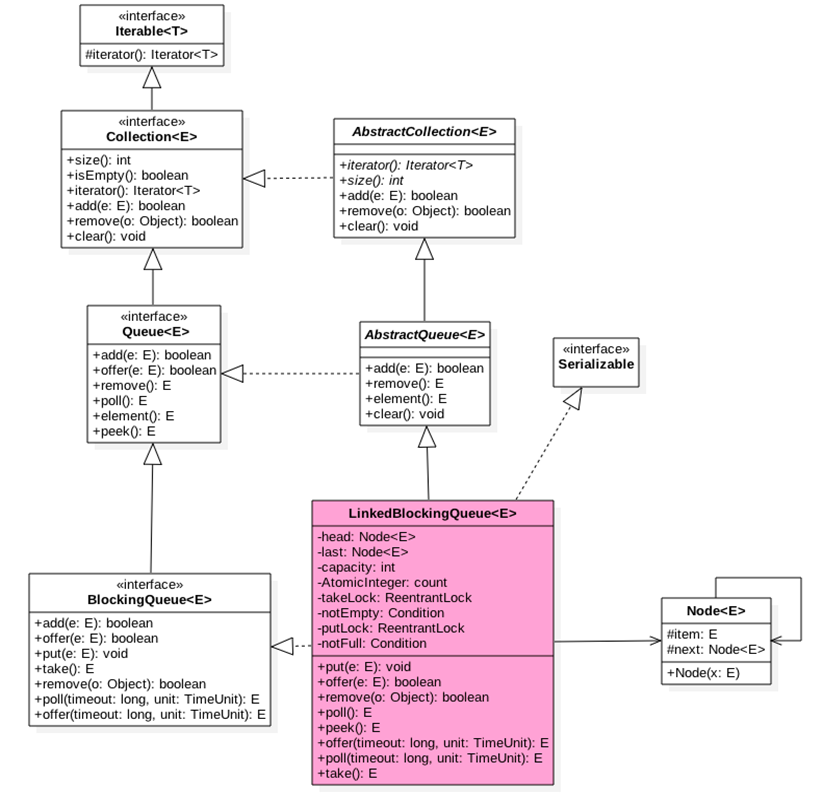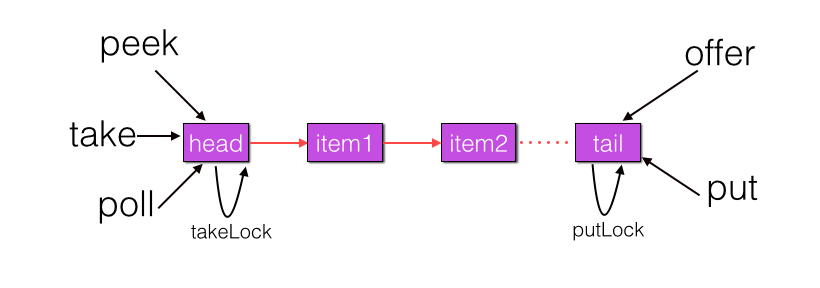#3
3
01. 云栖社区>
2. 博客>
3. 正文putLock控制同时只能有一个线程可以获取锁去添加元素，其他线程必须等待。另外notEmpty 和 notFull 是信号量，内部分别有一个条件队列用来存放进队和出队的时候被阻塞的线程，

```　　/** 执行take, poll等操作时候需要获取该锁 */
private final ReentrantLock takeLock = new ReentrantLock();

/** 当队列为空时候执行出队操作（比如take）的线程会被放入这个条件队列进行等待 */
private final Condition notEmpty = takeLock.newCondition();

/** 执行put, offer等操作时候需要获取该锁*/
private final ReentrantLock putLock = new ReentrantLock();

/**当队列满时候执行进队操作（比如put)的线程会被放入这个条件队列进行等待 */
private final Condition notFull = putLock.newCondition();

/** 当前队列元素个数 */
private final AtomicInteger count = new AtomicInteger(0);```

```public static final int   MAX_VALUE = 0x7fffffff;

this(Integer.MAX_VALUE);
}

if (capacity <= 0) throw new IllegalArgumentException();
this.capacity = capacity;
//初始化首尾节点,指向哨兵节点
last = head = new Node<E>(null);
}```

1.offer操作，向队列尾部插入一个元素，如果队列有空闲容量则插入成功后返回true，如果队列已满则丢弃当前元素然后返回false，如果 e元素为null，则抛出空指针异常(NullPointerException )，还有一点就是，该方法是非阻塞的。源码如下：

```public boolean offer(E e) {

//（1）空元素抛空指针异常
if (e == null) throw new NullPointerException();

//(2) 如果当前队列满了则丢弃将要放入的元素，然后返回false
final AtomicInteger count = this.count;
if (count.get() == capacity)
return false;

//(3) 构造新节点，获取putLock独占锁
int c = -1;
Node<E> node = new Node<E>(e);
final ReentrantLock putLock = this.putLock;
putLock.lock();
try {
//(4)如果队列不满则进队列，并递增元素计数
if (count.get() < capacity) {
enqueue(node);
c = count.getAndIncrement();
//(5)
if (c + 1 < capacity)
notFull.signal();
}
} finally {
//(6)释放锁
putLock.unlock();
}
//(7)
if (c == 0)
signalNotEmpty();
//(8)
return c >= 0;
}

private void enqueue(Node<E> node) {
last = last.next = node;
}```

```private void signalNotEmpty() {
final ReentrantLock takeLock = this.takeLock;
takeLock.lock();
try {
notEmpty.signal();
} finally {
takeLock.unlock();
}
}```

offer的总结：offer方法中通过使用putLock锁保证了在队尾新增元素的原子性和队列元素个数的比较和递增操作的原子性。

2.put操作，向队列尾部插入一个元素，如果队列有空闲则插入后直接返回true,如果队列已经满则阻塞当前线程知道队列有空闲插入成功后返回true，如果在阻塞的时候被其他线程设置了中断标志，

```　　public void put(E e) throws InterruptedException {
//（1）空元素抛空指针异常
if (e == null) throw new NullPointerException();
//(2) 构建新节点，并获取独占锁putLock
int c = -1;
Node<E> node = new Node<E>(e);
final ReentrantLock putLock = this.putLock;
final AtomicInteger count = this.count;
putLock.lockInterruptibly();
try {
//(3)如果队列满则等待
while (count.get() == capacity) {
notFull.await();
}
//（4）进队列并递增计数
enqueue(node);
c = count.getAndIncrement();
//(5)
if (c + 1 < capacity)
notFull.signal();
} finally {
//(6)
putLock.unlock();
}
//(7)
if (c == 0)
signalNotEmpty();
}```

3.poll操作，从队列头部获取并移除一个元素，如果队列为空则返回 null，该方法是不阻塞的。源码如下：

```　　public E poll() {
//(1)队列为空则返回null
final AtomicInteger count = this.count;
if (count.get() == 0)
return null;
//(2)获取独占锁
E x = null;
int c = -1;
final ReentrantLock takeLock = this.takeLock;
takeLock.lock();
try {
//(3)队列不空则出队并递减计数
if (count.get() > 0) {//3.1
x = dequeue();//3.2
c = count.getAndDecrement();//3.3
//(4)
if (c > 1)
notEmpty.signal();
}
} finally {
//(5)
takeLock.unlock();
}
//(6)
if (c == capacity)
signalNotFull();
//(7)返回
return x;
}
private E dequeue() {
Node<E> first = h.next;
h.next = h; // help GC
E x = first.item;
first.item = null;
return x;
}```

```　　private void signalNotFull() {
final ReentrantLock putLock = this.putLock;
putLock.lock();
try {
notFull.signal();
} finally {
putLock.unlock();
}
}```

poll 代码逻辑比较简单，值得注意的是获取元素时候只操作了队列的头节点。

4.peek 操作，获取队列头部元素但是不从队列里面移除，如果队列为空则返回 null，该方法是不阻塞的。源码如下：

```　　public E peek() {
//(1)
if (count.get() == 0)
return null;
//(2)
final ReentrantLock takeLock = this.takeLock;
takeLock.lock();
try {
//(3)
if (first == null)
return null;
else
//(4)
return first.item;
} finally {
//(5)
takeLock.unlock();
}
}```

5.take 操作，获取当前队列头部元素并从队列里面移除，如果队列为空则阻塞调用线程。如果队列为空则阻塞当前线程知道队列不为空，然后返回元素，如果在阻塞的时候被其他线程设置了中断标志，则被阻塞线程会抛出InterruptedException 异常而返回。源码如下：

```　　public E take() throws InterruptedException {
E x;
int c = -1;
final AtomicInteger count = this.count;
//(1)获取锁
final ReentrantLock takeLock = this.takeLock;
takeLock.lockInterruptibly();
try {
//(2)当前队列为空则阻塞挂起
while (count.get() == 0) {
notEmpty.await();
}
//(3)出队并递减计数
x = dequeue();
c = count.getAndDecrement();
//(4)
if (c > 1)
notEmpty.signal();
} finally {
//(5)
takeLock.unlock();
}
//(6)
if (c == capacity)
signalNotFull();
//(7)
return x;
}```

6.remove操作，删除队列里面指定元素，有则删除返回 true，没有则返回 false，源码如下：

```public boolean remove(Object o) {
if (o == null) return false;

//（1）双重加锁
fullyLock();
try {

//（2)遍历队列找则删除返回true
for (Node<E> trail = head, p = trail.next;
p != null;
trail = p, p = p.next) {
//(3)
if (o.equals(p.item)) {
return true;
}
}
//(4)找不到返回false
return false;
} finally {
//(5)解锁
fullyUnlock();
}
}```

```void fullyLock() {
putLock.lock();
takeLock.lock();
}```

```　　void unlink(Node<E> p, Node<E> trail) {
p.item = null;
trail.next = p.next;
if (last == p)
last = trail;
如果当前队列满，删除后，也不忘记唤醒等待的线程
if (count.getAndDecrement() == capacity)
notFull.signal();
}```

```void fullyUnlock() {
takeLock.unlock();
putLock.unlock();
}```

7.size操作，获取当前队列元素个数。源码如下：

```public int size() {
return count.get();
}```+ 关注